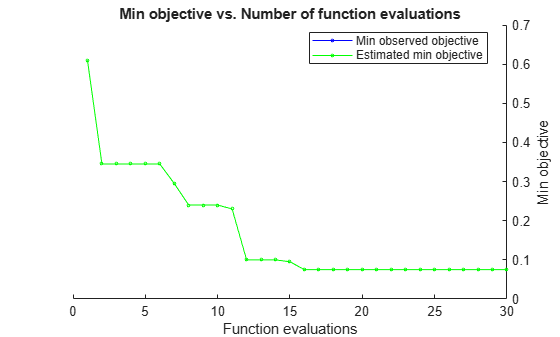# predictObjectiveEvaluationTime

Predict objective function run times at a set of points

## Syntax

``time = predictObjectiveEvaluationTime(results,XTable)``

## Description

example

````time = predictObjectiveEvaluationTime(results,XTable)` returns estimated objective evaluation times at the points in `XTable`.```

## Examples

collapse all

This example shows how to estimate the objective function evaluation time in an optimized Bayesian model of SVM classification.

Create an optimized SVM model. For details of this model, see Optimize a Cross-Validated SVM Classifier Using bayesopt.

```rng default grnpop = mvnrnd([1,0],eye(2),10); redpop = mvnrnd([0,1],eye(2),10); redpts = zeros(100,2); grnpts = redpts; for i = 1:100 grnpts(i,:) = mvnrnd(grnpop(randi(10),:),eye(2)*0.02); redpts(i,:) = mvnrnd(redpop(randi(10),:),eye(2)*0.02); end cdata = [grnpts;redpts]; grp = ones(200,1); grp(101:200) = -1; c = cvpartition(200,'KFold',10); sigma = optimizableVariable('sigma',[1e-5,1e5],'Transform','log'); box = optimizableVariable('box',[1e-5,1e5],'Transform','log'); minfn = @(z)kfoldLoss(fitcsvm(cdata,grp,'CVPartition',c,... 'KernelFunction','rbf','BoxConstraint',z.box,... 'KernelScale',z.sigma)); results = bayesopt(minfn,[sigma,box],'IsObjectiveDeterministic',true,... 'AcquisitionFunctionName','expected-improvement-plus','Verbose',0);```Predict the evaluation time for various points.

```sigma = logspace(-5,5,11)'; box = 1e5*ones(size(sigma)); XTable = table(sigma,box); time = predictObjectiveEvaluationTime(results,XTable); [XTable,table(time)]```
```ans=11×3 table sigma box time ______ _____ _______ 1e-05 1e+05 0.23532 0.0001 1e+05 0.23431 0.001 1e+05 0.23457 0.01 1e+05 0.23901 0.1 1e+05 0.2714 1 1e+05 0.51959 10 1e+05 1.2671 100 1e+05 0.91673 1000 1e+05 0.40551 10000 1e+05 0.25126 1e+05 1e+05 0.21108 ```

## Input Arguments

collapse all

Bayesian optimization results, specified as a `BayesianOptimization` object.

Prediction points, specified as a table with D columns, where D is the number of variables in the problem. The function performs its predictions on these points.

Data Types: `table`

## Output Arguments

collapse all

Estimated objective evaluation times, returned as an `N`-by-`1` vector, where `N` is the number of rows of `XTable`. The estimated values are the means of the posterior distribution of the Gaussian process model of the evaluation times of the objective function.